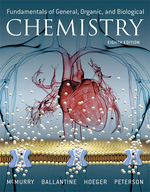×
Get Full Access to Fundamentals Of General, Organic, And Biological Chemistry (Mastering Chemistry) - 8 Edition - Chapter 3 - Problem 3.55
Get Full Access to Fundamentals Of General, Organic, And Biological Chemistry (Mastering Chemistry) - 8 Edition - Chapter 3 - Problem 3.55

×ISBN: 9780134015187 2044

## Solution for problem 3.55 Chapter 3

Fundamentals of General, Organic, and Biological Chemistry (Mastering Chemistry) | 8th Edition

• Textbook Solutions
• 2901 Step-by-step solutions solved by professors and subject experts
• Get 24/7 help from StudySoup virtual teaching assistantsFundamentals of General, Organic, and Biological Chemistry (Mastering Chemistry) | 8th Edition

4 5 1 329 Reviews
28
1
Problem 3.55

Write the electron configurations of $$\mathrm{Co},\mathrm{Co}^{2+}$$, and $$\mathrm{Co}^{3+}$$.

Text Transcription:

Co/Co^2+

Co^3+

Step-by-Step Solution:
Step 1 of 3

Principles of Chemistry: A molecular approach Chapter 2 Imaging and moving  Gerd, Binning and Heinrich Roher -- experiment with electric current over a flat metal surface = tunneling current to view atoms  Law of conservation of matter o Matter can neither created or destroyed  Total reactant = total product  Dalton - English chemist o Atomic theory of matter o Atoms are small, discrete, indivisible pieces of matter o Elements are made up of atoms o All atoms are the same  Law of definite proportions o Same thing every time in same proportions  Example: NaCl is always a 1:1 proportions  Law of multiple proportions o 2 elements A and X can form different compounds when made in different proportions  Ex: H2O and H2O2 or CO and CO2 Discovery of E-  Thomson's charge to mass experiments with cathode rays o He believed these particles were building blocks of matter o Cathode ray particles = E- Atoms  JJ Thomas and the Plum pudding model o Atoms are composed of a positive cloud of matter which e- are embedded in o This structure does not hold up today  Rutherford o Gold foil experiment o Led to Discovery of the nucleus o Beam would go straight through the solid but some particles would veer off to the side  It w

Step 2 of 3

Step 3 of 3

##### ISBN: 9780134015187

Fundamentals of General, Organic, and Biological Chemistry (Mastering Chemistry) was written by Aimee Notetaker and is associated to the ISBN: 9780134015187. This full solution covers the following key subjects: . This expansive textbook survival guide covers 281 chapters, and 240 solutions. The answer to “?Write the electron configurations of $$\mathrm{Co},\mathrm{Co}^{2+}$$, and $$\mathrm{Co}^{3+}$$.Text Transcription:Co/Co^2+Co^3+” is broken down into a number of easy to follow steps, and 9 words. This textbook survival guide was created for the textbook: Fundamentals of General, Organic, and Biological Chemistry (Mastering Chemistry), edition: 8. Since the solution to 3.55 from 3 chapter was answered, more than 206 students have viewed the full step-by-step answer. The full step-by-step solution to problem: 3.55 from chapter: 3 was answered by Aimee Notetaker, our top Chemistry solution expert on 04/25/22, 03:57PM.

## Discover and learn what students are asking

Calculus: Early Transcendental Functions : Preparation for Calculus
?In Exercises 1–4, find any intercepts. $$y=(x-3) \sqrt{x+4}$$

Statistics: Informed Decisions Using Data : Inference about the Difference between Two Medians: Dependent Samples
?Reaction-Time Experiment Researchers at the University of Mississippi wanted to learn the reaction times of students to different stimuli. In the foll

Unlock Textbook Solution